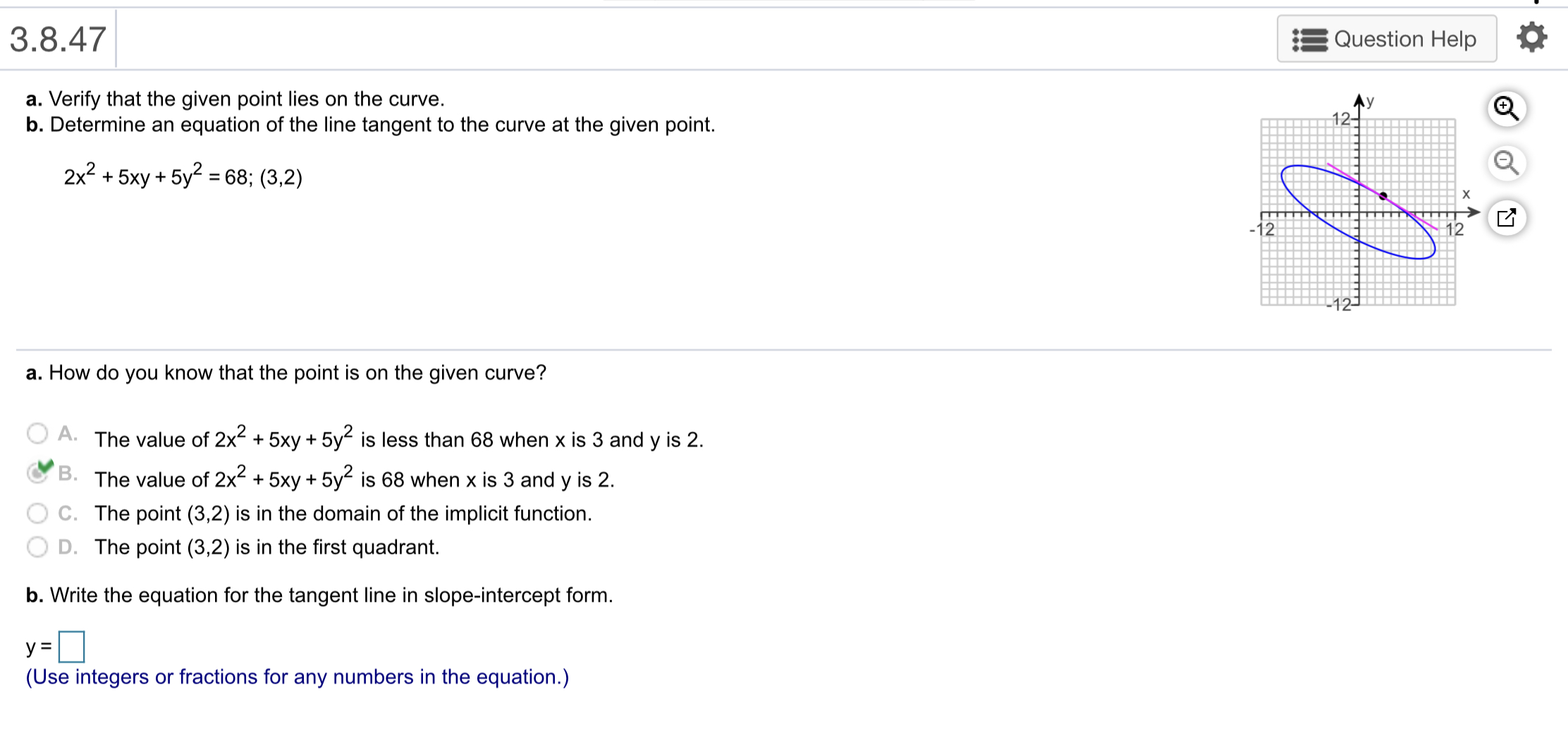# 3.8.47 Question Help a. Verify that the given point lies on the curve. b. Determine an equation of the line tangent to the curve at the given point. 12 2x2+5xy+5y -68; (3.2) 12 a. How do you know that the point is on the given curve? A. The value of 2x2 +5xy +5y2 is less than 68 when x is 3 and y is 2. The value of 2x+5xy + 5y is 68 when x is 3 and y is 2 C. The point (3,2) is in the domain of the implicit function. D. The point (3,2) is in the first quadrant. b. Write the equation for the tangent line in slope-intercept form (Use integers or fractions for any numbers in the equation.)

Questionhelp_outlineImage Transcriptionclose3.8.47 Question Help a. Verify that the given point lies on the curve. b. Determine an equation of the line tangent to the curve at the given point. 12 2x2+5xy+5y -68; (3.2) 12 a. How do you know that the point is on the given curve? A. The value of 2x2 +5xy +5y2 is less than 68 when x is 3 and y is 2. The value of 2x+5xy + 5y is 68 when x is 3 and y is 2 C. The point (3,2) is in the domain of the implicit function. D. The point (3,2) is in the first quadrant. b. Write the equation for the tangent line in slope-intercept form (Use integers or fractions for any numbers in the equation.) fullscreen

### Want to see this answer and more?

Experts are waiting 24/7 to provide step-by-step solutions in as fast as 30 minutes!*

*Response times vary by subject and question complexity. Median response time is 34 minutes and may be longer for new subjects.
Tagged in
MathCalculus

### Derivative